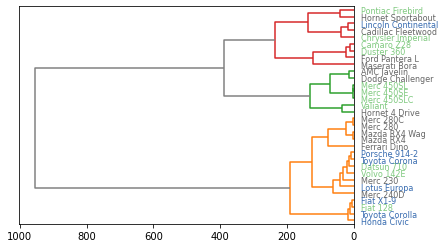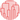# Color dendrogram labels

You can find how to build a dendrogram and customise most of its features in the posts #400 and #401. In this post, you will see how to change colors of labels in a dendrogram.

After clustering your data and plotting a dendrogram, you probably want to compare the structure you get with your expectations. You can make this comparison by coloring labels according to your expectation.
In this example, mtcars dataset is used. It is a numeric matrix that gives the features of cars. We can cluster these cars, and represent their structure in a group by using the `dendrogram()` function of the scipy library of python. In the example, you will see how to color the labels(car names) according to their cylinder(the ‘cyl’ column). By coloring according to cylinder feature, it is possible to see visually if the cylinder is responsible of this structure!

``````# Libraries
import pandas as pd
from matplotlib import pyplot as plt
from scipy.cluster.hierarchy import dendrogram, linkage
import numpy as np

# Data set
url = 'https://python-graph-gallery.com/wp-content/uploads/mtcars.csv'
df = pd.read_csv(url)
df = df.set_index('model')

# Calculate the distance between each sample
Z = linkage(df, 'ward')

# Make the dendrogram
dendrogram(Z, labels=df.index, leaf_rotation=0, orientation="left", color_threshold=240, above_threshold_color='grey')

# Create a color palette with 3 colors for the 3 cyl possibilities
my_palette = plt.cm.get_cmap("Accent", 3)

# transforme the 'cyl' column in a categorical variable. It will allow to put one color on each level.
df['cyl']=pd.Categorical(df['cyl'])
my_color=df['cyl'].cat.codes

# Apply the right color to each label
ax = plt.gca()
xlbls = ax.get_ymajorticklabels()
num=-1
for lbl in xlbls:
num+=1
val=my_color[num]
lbl.set_color(my_palette(val))

# Show the graph
plt.show()``````## Contact & Edit

👋 This document is a work by Yan Holtz. Any feedback is highly encouraged. You can fill an issue on Github, drop me a message onTwitter, or send an email pasting `yan.holtz.data` with `gmail.com`.

This page is just a jupyter notebook, you can edit it here. Please help me making this website better 🙏!